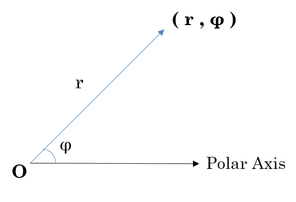Python Little Goal 11

Python Day 17: Polar Coordinate in Python

” You are round and short,
.

.

in polar coordinate.”

So today we will introduce the most basic calculation in Python for the Polar coordinates.

z= x+ y*i (x, y in R, i in C)Output format:

first line τ: distance from z to origin.

second line φ: counterclockwise angle measure from the positive x-axis to the line segment that joins z to the origin.Noel_Bauza / Pixabay

Code:

import cmath
a=cmath.polar(complex(1.0,2.0))
print(a,sep="\")

Output:

(2.23606797749979, 1.1071487177940904)

Polar Plotimport numpy as np
import matplotlib.pyplot as plt

# Fixing random state for reproducibility
np.random.seed(19680801)

# Compute areas and colors
N = 150
r = 2 * np.random.rand(N)
theta = 2 * np.pi * np.random.rand(N)
area = 200 * r**2
colors = theta

fig = plt.figure()
ax = fig.add_subplot(111, projection='polar')
c = ax.scatter(theta, r, c=colors, s=area, cmap='hsv', alpha=0.75)

Happy Practicing!

Reference:

https://www.hackerrank.com/challenges/polar-coordinates/problem

https://matplotlib.org/3.1.0/gallery/pie_and_polar_charts/polar_scatter.html

This site uses Akismet to reduce spam. Learn how your comment data is processed.

Social media & sharing icons powered by UltimatelySocial